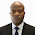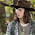## Monday, July 10, 2017

Eurocode 2 uses the variable strut inclination method for shear design. It is slightly more complex than the procedure in BS 8110 but can result in savings in the amount of shear reinforcement required. Like BS 8110, EC2 models shear behaviour on the truss analogy
in which the concrete acts as the diagonal struts, the stirrups act as the vertical ties, the tension reinforcement forms the bottom chord, and the compression steel/ concrete forms the top chord. Whereas in BS 8110 the strut angle has a fixed value of 45°, in EC2 it can vary between 21.8° and 45° and it is this feature which is responsible for possible reductions in the volume of shear reinforcement.

SOLVED EXAMPLE
For the beam loaded as shown below and disregarding load factors, we are going to obtain the shear reinforcement required for section A. The shear force at the centreline of support has been adopted for this design, and the area of tension steel provided at this section As1 = 4825 mm2.

Design Data:
Concrete Strength = 35 N/mm2
Grade of steel = 460 N/mm2
Width of beam = 400mm
Depth of beam = 600mm
Effective depth = 543mm
Area of tension steel provided at section As1 = 4825 mm2

SHEAR DESIGN ACCORDING EC2
Support A; VEd = 500.46 KN

VRd,c = [CRd,c.k.(100ρ1 fck)(1/3) + k1cp]bw.d ≥ (Vmin + k1cp) bw.d

CRd,c = 0.18/γc = 0.18/1.5 = 0.12
k = 1 + √(200/d) = 1 + √(200/543) = 1.606 > 2.0, therefore, k = 1.606
Vmin = 0.035k(3/2) fck0.5
Vmin = 0.035 × (1.606)1.5 × 350.5 = 0.421 N/mm2
ρ1 = As/bd = 4825/(400 × 543) = 0.022 > 0.02; Therefore take 0.02

σcp = NEd/Ac < 0.2fcd (Where NEd is the axial force at the section, Ac = cross sectional area of the concrete), fcd = design compressive strength of the concrete.) Take NEd = 0

VRd,c = [0.12 × 1.606 (100 × 0.02 × 35 )(1/3)] 400 × 543 = 172511.992 N = 172.511 KN

Since VRd,c (172.511 KN) < VEd (500.46 KN), shear reinforcement is required.
The compression capacity of the compression strut (VRd,max) assuming θ = 21.8° (cot θ = 2.5)

VRd,max = (bw.z.v1.fcd) / (cot⁡θ + tanθ)
V1 = 0.6(1 - fck/250) = 0.6(1 - 35/250) = 0.516
fcd = (αcc fck) / γc = (0.85 × 35) / 1.5 = 19.833 N/mm2
Let z = 0.9d

VRd,max = [(400 × 0.9 × 543 × 0.516 × 19.833) / (2.5 + 0.4)] × 10-3 = 689.83 KN

Since VRd,c < VEd < VRd,max

Hence Asw / S = VEd / (0.87 Fyk z cot θ) = 500460 / (0.87 × 460 × 0.9 × 543 × 2.5 ) = 1.0235

Minimum shear reinforcement;
Asw / S = ρw,min × bw × sinα (α = 90° for vertical links)
ρw,min = (0.08 × √(Fck)) / Fyk = (0.08 × √35) / 460 = 0.001028
Asw/Smin = 0.001028 × 400 × 1 = 0.411
Maximum spacing of shear links = 0.75d = 0.75 × 543 = 407.25

Provide X10mm @ 150mm c/c as shear links (Asw/S = 1.0467) Ok!!!!

SHEAR DESIGN BY BS 8110-1:1997
Design of support A

Ultimate shear force at centerline of support
V = 500.46 KN

Using the shear force at the centreline of support;
Shear stress v = V/(bd ) = (500.46 × 103) / (400 × 543) = 2.304 N/mm2

v(2.304 N/mm2 ) < 0.8√Fcu (4.732 N/mm2 ). Hence, dimensions of the cross-section is adequate for shear.

Concrete resistance shear stress
vc = 0.632 × (100As/bd)1/3 (400/d)1/4

(100As/bd) = (100 × 4825) / (400 × 543) = 2.221 < 3 (See Table 3.8 BS 8110-1;1997)
(400/d)1/4 = (400/543)1/4 = 0.926; But for members with shear reinforcement, this value should not be less than 1. Therefore take value as 1.0

vc = 0.632 × (2.221)1/3 × 1.0 = 0.8245 N/mm2

For Fcu = 35 N/mm2, vc = 0.8245 × (35/25)1/3 = 0.922 N/mm2

Let us check;
(Vc + 0.4)1.322 N/mm2 < v(2.304 N/mm2) < 0.8√Fcu(4.732 N/mm2)

Let us try 2 legs of Y10mm bars (Area of steel provided = 157 mm2

Asv/Sv = (0.95 × Fyv) / (bv (v - vc)) = [400 × (2.304 - 0.922)] / (0.95 × 460) = 1.264

Maximum spacing = 0.75d = 0.75 × 543 = 407.25 mm
Provide Y10mm @ 100 mm c/c links as shear reinforcement (Asv/Sv = 1.57)

We can therefore see that disregarding load factor and flexural design requirements, EC2 is more economical than BS 8110 in shear design, and in this case study by about 19%.

1.Good job engineer. Make it more elaborate next time so that one can follow the steps all the way without the need for a textbook on the side. God bless you.

1.Thank you. This is more of a solved example. If you need more understanding on basic design theories and procedures, you can contact me directly.

I may also post on that in the nearest future. God bless.

2.• Just wanted to let you know and say very great job on your blog. I for one agree with what you are saying and hope to see more of your posts in the near future. BIM Consulting
BIM Solutions
BIM Implementation
Point Cloud to BIM conversion

3.How to get different 19% between design shear reinforced BS vs EU ?